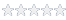# Calculating time diffrerence using DateTime data type

#### Knowledge Base Article # Q200083

Summary How to calculate time difference between two DateTime valuesLogin to rate article
ATEasy's DateTime type values are really a Double data type - the same applies to a DateTime value in a variant (vtDate) type.
The integer part of the double number is the number of days since 12-30-1899.  The fractional part is effectively the time-of-day.
The easiest way to convert a date string into our DateTime is to assign the string to a variant and then assign the variant to a DateTime variable, for example:

vr01 = "8/25/2006 17:49"
dt01 = vr01

DateTime values can be compared with the expected meaning.  Since they are really Double values, if you subtract them and store the result in a Double, you have the number of days between the two dates.  Similarly, adding a Double to a DateTime, adjusts the date and time by that many days plus a fraction of a day.
Here is an example of subtracting two day/time:

vr1:Variant
vr2:Variant

vr1="08/27/06 "
print VarChangeType(vr1, vtDate)
print vr1
vr2="08/28/06 10:48"
print VarChangeType(vr2, vtDate)
print vr2
print vr2-vr1

The example will print:

0
8/27/2006
0
8/28/2006 10:48:00 AM
1.44999999999709 (one day and .449 of 24 hours)
Article Date 3/8/2007
Keywords ATEasy, DateTime, vtDate, Variant

 Login to rate article>